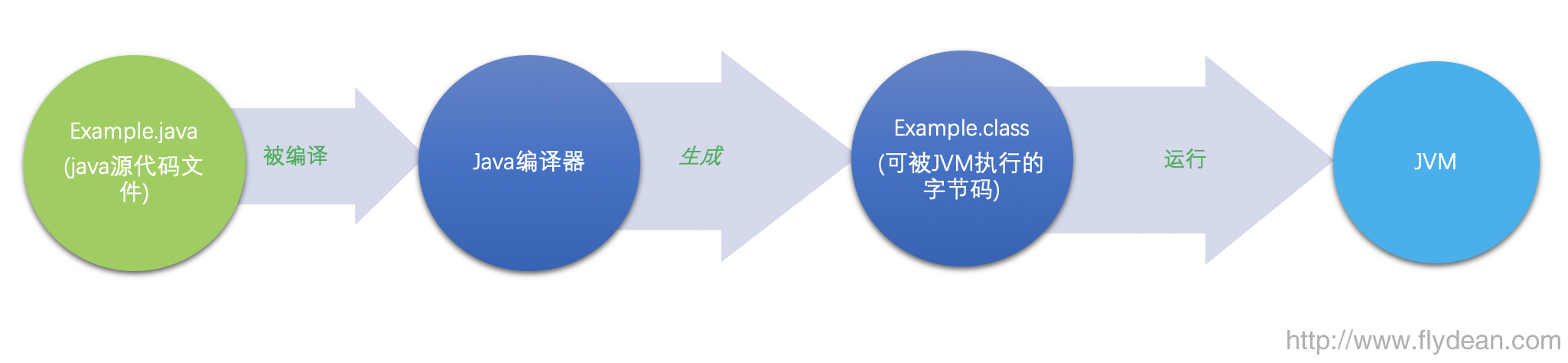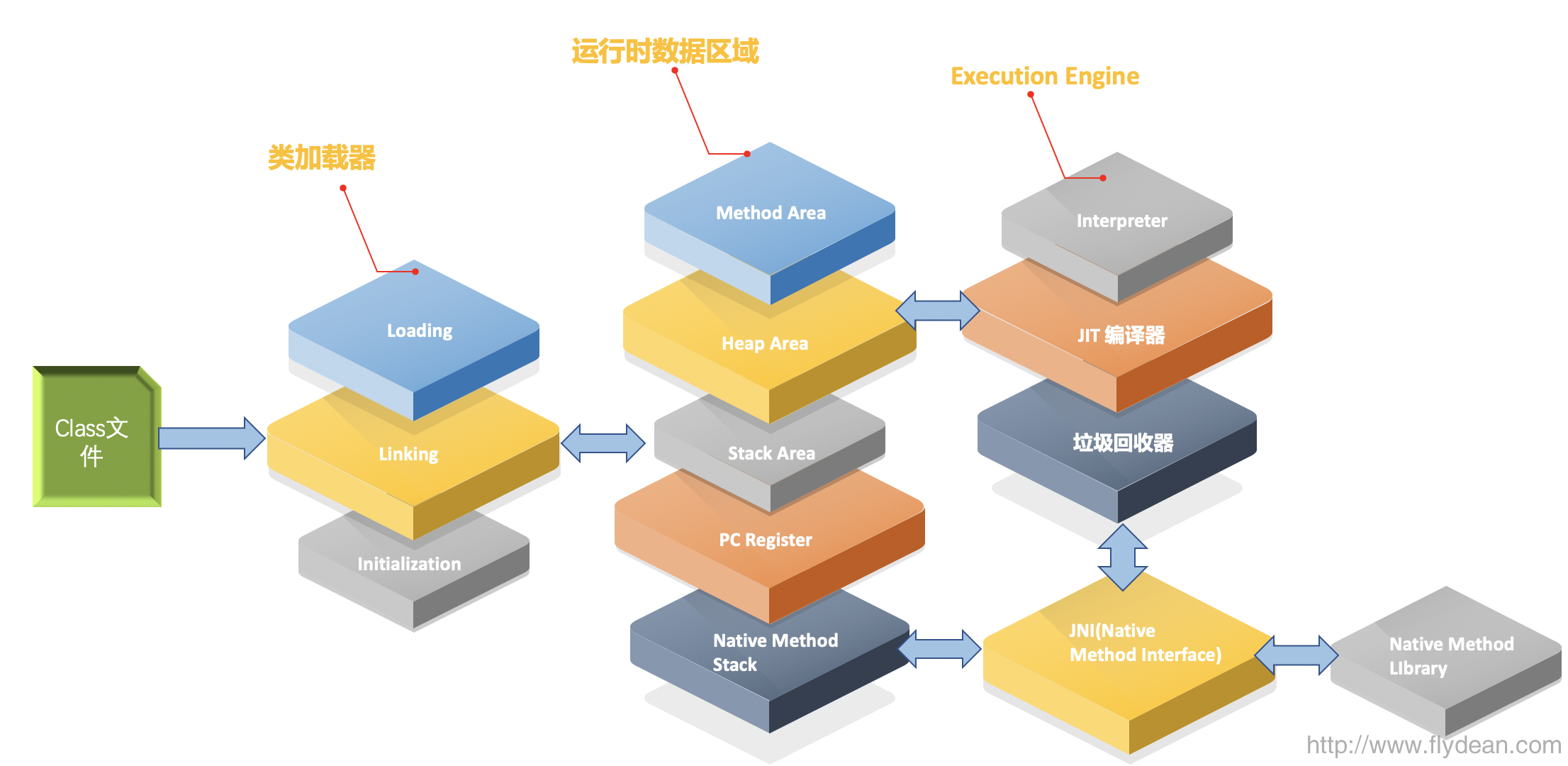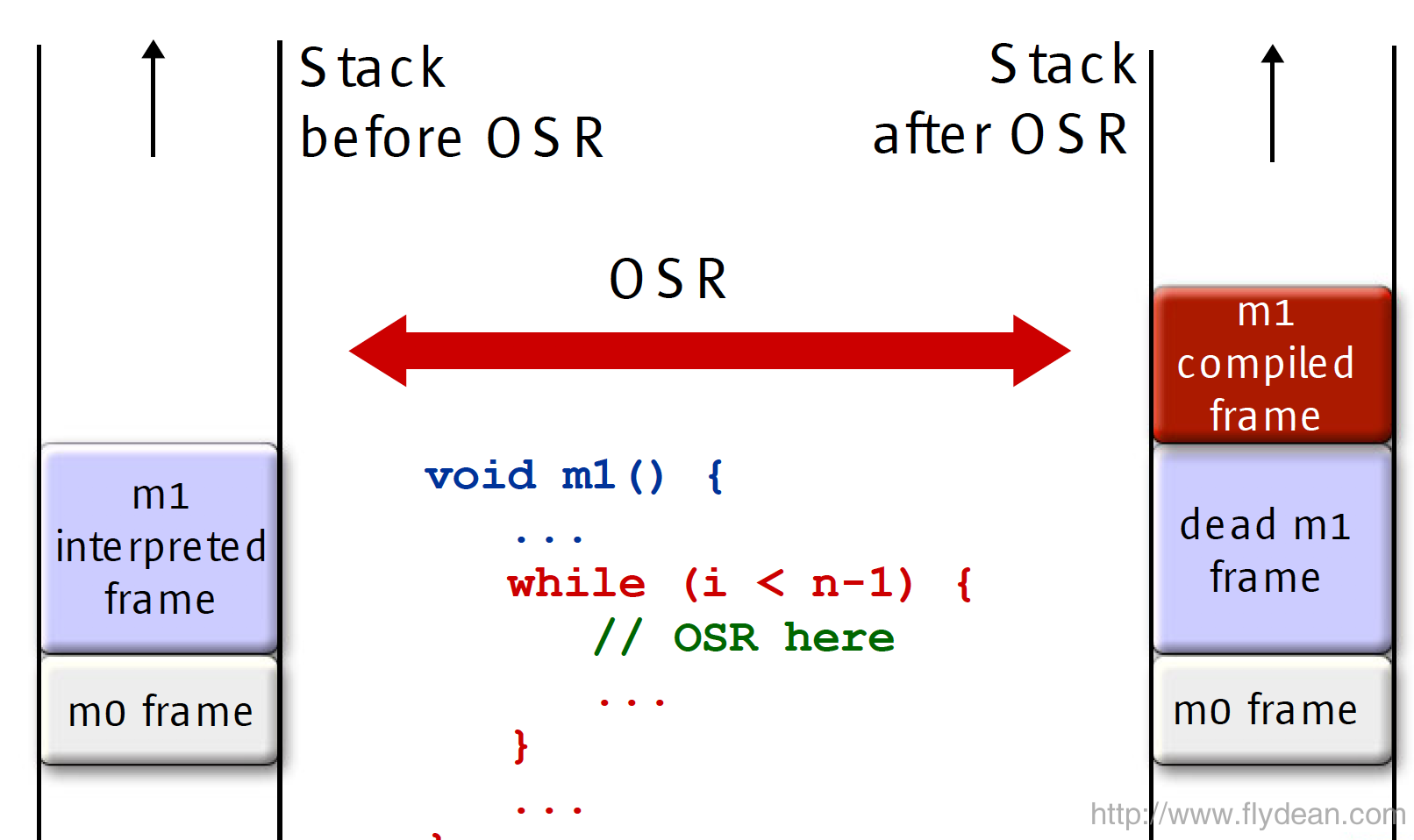# 小师妹学 JVM 之: 深入理解 JIT 和编译优化 - 你看不懂系列# JIT编译器# Tiered Compilation分层编译

Server端的JIT编译也不是立马进行的，它可能需要收集到足够多的信息之后，才进行编译。

1. 第一层就是禁用C1和C2编译器，这个时候没有JIT进行。

2. 第二层就是只开启C1编译器，因为C1编译器只会进行一些简单的JIT优化，所以这个可以应对常规情况。

3. 第三层就是同时开启C1和C2编译器。

-XX:+TieredCompilation

# OSR（On-Stack Replacement）1. 需要调试的情况

1. 代码废弃的情况

1. 优化之前编译的代码

# 常见的编译优化举例

## Inlining内联

int a = 1;int b = 2;int result = add(a, b);...public int add(int x, int y) { return x + y; }int result = a + b; //内联替换

## Branch Prediction分支预测

// make an array of random doubles 0..1double[] bigArray = makeBigArray();for (int i = 0; i < bigArray.length; i++){ double cur = bigArray[i]; if (cur > 0.5) { doThis();} else { doThat();}}

## Loop unswitching

  int i, w, x, y;  for (i = 0; i < 1000; i++) {    x[i] += y[i];    if (w)      y[i] = 0;  }

  int i, w, x, y;  if (w) {    for (i = 0; i < 1000; i++) {      x[i] += y[i];      y[i] = 0;    }  } else {    for (i = 0; i < 1000; i++) {      x[i] += y[i];    }  }

## Loop unrolling展开

 int x; for (x = 0; x < 100; x++) {     delete(x); }

 int x;  for (x = 0; x < 100; x += 5 ) {     delete(x);     delete(x + 1);     delete(x + 2);     delete(x + 3);     delete(x + 4); }

## Escape analysis逃逸分析

  public static void main(String[] args) {    example();  }  public static void example() {    Foo foo = new Foo(); //alloc    Bar bar = new Bar(); //alloc    bar.setFoo(foo);  }}class Foo {}class Bar {  private Foo foo;  public void setFoo(Foo foo) {    this.foo = foo;  }}

public String getNames() {     Vector<String> v = new Vector<>();     v.add("Me");     v.add("You");     v.add("Her");     return v.toString();}

Vector是一个同步对象，如果是在单线程环境中，这个同步锁是没有意义的，因此在JDK6之后，锁只在被需要的时候才会使用。

# 总结## 评论 (1 条评论)2020 年 06 月 17 日 06:42